Published: 30 September 2013

# Study of vibration characteristics of the short thin cylindrical shells and its experiment

Zhong Luo1
Ning Sun2
Yu Wang3
Kai Zhang4
Qingkai Han5
1, 2, 4School of Mechanical Engineering & Automation, Northeastern University, China
3School of Mechanical Engineering and Automation University of Science and Technology Liaoning, China
5School of Mechanical Engineering, Dalian University of Technology, China
Corresponding Author:
Zhong Luo
Views 129

#### Abstract

The short thin cylindrical shells are important component used in rotating machinery and its function is to connect shafts and transmitted torque. The kind of components is always destroyed due to vibrational state, so it is necessary to further research on the vibration characteristics. In this paper, the vibration characteristics of short thin cylindrical shells are solved using the beam function method, the transfer matrix method and the finite element method respectively. The solving results of three calculating methods are compared by simulation in the clamped-free and clamped-clamped boundary conditions. The simulation results show that the solving results of the transfer matrix method are close to the results of finite element method, but the deviation of the results of the beam functions method is larger than the other two methods. Furthermore, the experiments of the short thin cylindrical shell in the clamped-free boundary conditions are studied. The experimental results verify that the transfer matrix method and the finite element method are applicability to solve the vibration characteristics of the short thin cylindrical shells.

## 1. Introduction

Short thin cylindrical shell usually means that the axial half-wave number is 1, and the ratios of thickness and other minimum parameter (i.e. diameter and length) is between 1/80 and 1/5 . The short thin cylindrical shell has grown in importance with the increasing use of shell structures for a wide variety of applications in aerospace, shipbuilding, chemical machinery and other branches of engineering. It is important component used in rotating machinery and its function is to connect shafts and transmitted torque. It is subjected to centrifugal force, aerodynamic force, and vibration alternating force and other load during its working. The kind of components is always destroyed due to vibrational state. So it is necessary to further research on the vibration characteristics.

Currently numerous studies have been made to understand the vibration characteristic of the short thin cylindrical shell. In order to calculate the inherent characteristic of cantilever cylindrical shell, the Rayleigh-Ritz method, the axis-symmetry finite element method and the exact analytic method are employed by C. B. Sharma and D. J. Johns [2-3]. By using of the Love’s shell equations, the influence of boundary conditions for a thin rotating stiffened cylindrical shell is researched by Lee . Vibration characteristics of the laminated cylindrical shell are studied, then influence of boundary conditions and geometric parameters for system dynamics characteristics is analyzed by Zhang . Studies for dynamic characteristic of boundary conditions for thin cylindrical shells have also been carried out by Lam and Loy . Li Xuebin presents a new approach of separation of variables in circular cylindrical shell analysis for arbitrary boundary conditions . The instant response of the laminated shell is subject to radial impacting loads and axial pressure load, and the boundary condition investigated in the study is clamped-simply. The analysis is carried out using Love-type shell theory and the first order shear deformation theory by Jafari and Hamid [8, 9]. However, many of these studies are restricted to theory analyses. Studies for the contrast test of different analyze methods are seldom attempted, especially the experimental tests.

In this paper, the vibration characteristics of short thin cylindrical shells are solved using three analytical methods which is the beam function method, the transfer matrix method and the finite element method respectively, and then using the results of experiment confirmed the correctness and validity of analysis methods.

## 2. Analysis based on the beam function method

Figure 1 shows the nomenclature of a short thin cylindrical shell. The reference surface of the cylinder is taken at the middle surface where an orthogonal coordinate system ($Ox\theta z$) is fixed. The coordinates system ($Ox\theta z$) is the coordinates system ($O\text{'}x\text{'}\theta \text{'}r$) translate $R$ from origin $O$ along $r$ direction to one point of the middle surface. $x$-axis and ${x}^{\text{'}}$-axis are parallel and in the same direction. $\theta$-axis and ${\theta }^{\text{'}}$-axis are overlapping and in the same direction. $z$-axis and $r$-axis are overlapping. In the Figure 1, $R$ is the radius, $L$ is the length, $h$ is the thickness, $\rho$ is the material density, $E$ is the Young's modulus and $\mu$ is the Poisson's ratio. The deformations of the cylindrical shell in the $x$, $\theta$ and $z$ directions are denoted by $u\left(x,\theta ,t\right)$, $v\left(x,\theta ,t\right)$ and $w\left(x,\theta ,t\right)$, respectively.

Fig. 1The structures of the thin-walled cylindrical shells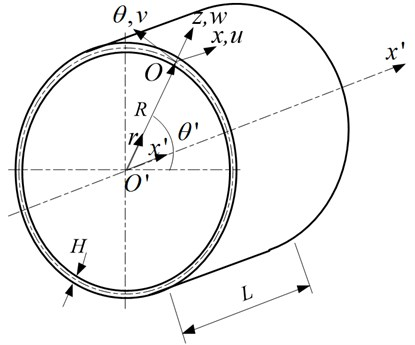### 2.1. Dynamic functions

The dynamic functions for a short thin cylindrical shell which describe the vibration characteristics of the vertical, tangential and radial vibrate can be written as :

1
$\rho H\frac{{\partial }^{2}u}{\partial {t}^{2}}=K\left(\frac{{\partial }^{2}u}{\partial {x}^{2}}+\frac{1-\mu }{2{R}^{2}}\frac{{\partial }^{2}u}{\partial {\theta }^{2}}+\frac{1+\mu }{2R}\frac{{\partial }^{2}v}{\partial x\partial \theta }+\frac{\mu }{R}\frac{\partial w}{\partial x}\right),$
2
$\rho H\frac{{\partial }^{2}v}{\partial {t}^{2}}=K\left(\frac{1+\mu }{2R}\frac{{\partial }^{2}u}{\partial x\partial \theta }+\frac{1-\mu }{2}\frac{{\partial }^{2}v}{\partial {x}^{2}}+\frac{1}{{R}^{2}}\frac{{\partial }^{2}v}{\partial {\theta }^{2}}+\frac{1}{{R}^{2}}\frac{\partial w}{\partial \theta }\right)$
$+D\frac{1}{{R}^{2}}\left(\frac{\left(1-\mu \right)}{2}\frac{{\partial }^{2}v}{\partial {x}^{2}}+\frac{1}{{R}^{2}}\frac{{\partial }^{2}v}{\partial {\theta }^{2}}-\frac{{\partial }^{3}w}{\partial {x}^{2}\partial \theta }-\frac{1}{{R}^{2}}\frac{{\partial }^{3}w}{\partial {\theta }^{3}}\right),$
3
$\rho H\frac{{\partial }^{2}w}{\partial {t}^{2}}=D\left(\frac{1}{{R}^{4}}\frac{{\partial }^{3}v}{\partial {\theta }^{3}}\text{ }+\frac{1}{{R}^{2}}\frac{{\partial }^{3}v}{\partial {x}^{2}\partial \theta }-\frac{{\partial }^{4}w}{\partial {x}^{4}}-\frac{1}{{R}^{4}}\frac{{\partial }^{4}w}{\partial {\theta }^{4}}-\frac{2}{{R}^{2}}\frac{{\partial }^{4}w}{\partial {x}^{2}\partial {\theta }^{2}}\right)$
$-K\left(\frac{\mu }{R}\frac{\partial u}{\partial x}+\frac{1}{{R}^{2}}\frac{\partial v}{\partial \theta }+\frac{1}{{R}^{2}}w\right),$

where $K$ is the different membrane stiffness, $D$ is the flexural rigidity; $\rho H\frac{{\partial }^{2}u}{\partial {t}^{2}}$, $\rho H\frac{{\partial }^{2}v}{\partial {t}^{2}}$ and $\rho H\frac{{\partial }^{2}w}{\partial {t}^{2}}$ are inertia item.

In terms of the displacements $u$, $v$ and $w$ is derived for the free vibration of a rotating cylindrical shell and be written in the following form:

4
$\begin{array}{c}{L}_{11}u+{L}_{12}v+{L}_{13}w=0,\\ {L}_{21}u+{L}_{22}v+{L}_{23}w=0,\\ {L}_{31}u+{L}_{32}v+{L}_{33}w=0,\end{array}}$

where ${L}_{ij}\left(i,j=1,\text{\hspace{0.17em}}2,\text{\hspace{0.17em}}3\right)$ are differential operators and can be written as follows:

${L}_{11}=-\rho H\frac{{\partial }^{2}}{\partial {t}^{2}}+K\frac{{\partial }^{2}}{\partial {x}^{2}}+K\frac{1-\mu }{2{R}^{2}}\frac{{\partial }^{2}}{\partial {\theta }^{2}},$
${L}_{12}=K\frac{1+\mu }{2R}\frac{{\partial }^{2}}{\partial x\partial \theta },$
${L}_{13}=K\frac{\mu }{R}\frac{\partial }{\partial x},$
${L}_{21}=K\frac{1+\mu }{2R}\frac{{\partial }^{2}}{\partial x\partial \theta },$
${L}_{22}=-\rho H\frac{{\partial }^{2}}{\partial {t}^{2}}+K\frac{1-\mu }{2}\frac{{\partial }^{2}}{\partial {x}^{2}}\text{ }+K\frac{1}{{R}^{2}}\frac{{\partial }^{2}}{\partial {\theta }^{2}}+D\frac{\left(1-\mu \right)}{2{R}^{2}}\frac{{\partial }^{2}}{\partial {x}^{2}}+D\frac{1}{{R}^{4}}\frac{{\partial }^{2}}{\partial {\theta }^{2}},$
${L}_{23}=K\frac{1}{{R}^{2}}\frac{\partial }{\partial \theta }-D\frac{1}{{R}^{4}}\frac{{\partial }^{3}}{\partial {\theta }^{3}}-D\frac{1}{{R}^{2}}\frac{{\partial }^{3}}{\partial {x}^{2}\partial \theta },$
${L}_{31}=K\frac{\mu }{R}\frac{\partial }{\partial x},$
${L}_{32}=K\frac{1}{{R}^{2}}\frac{\partial }{\partial \theta }-D\frac{1}{{R}^{4}}\frac{{\partial }^{3}}{\partial {\theta }^{3}}-D\frac{1}{{R}^{2}}\frac{{\partial }^{3}}{\partial {x}^{2}\partial \theta },$
${L}_{33}=\rho H\frac{{\partial }^{2}}{\partial {t}^{2}}+K\frac{1}{{R}^{2}}+D\frac{{\partial }^{4}}{\partial {x}^{4}}+D\frac{1}{{R}^{4}}\frac{{\partial }^{4}}{\partial {\theta }^{4}}+D\frac{2}{{R}^{2}}\frac{{\partial }^{4}}{\partial {x}^{2}\partial {\theta }^{2}}.$

### 2.2. Analytical method

The vibration mode functions of short thin cylindrical shell are derived for the axial beam function and the circumferential triangle function and can be written in the following form :

5
$\begin{array}{l}u\left(x,\theta ,t\right)=\sum _{m=1}^{\infty }\sum _{n=1}^{\infty }{\stackrel{-}{U}}_{mn}{\Phi }_{u}\left(x,\theta ,t\right)=\sum _{m=1}^{\infty }\sum _{n=1}^{\infty }{\stackrel{-}{U}}_{mn}{\varphi }_{m}^{u}\left(x\right){\phi }_{n}^{u}\left(\theta ,t\right),\\ v\left(x,\theta ,t\right)=\sum _{m=1}^{\infty }\sum _{n=1}^{\infty }{\stackrel{-}{V}}_{mn}{\Phi }_{v}\left(x,\theta ,t\right)=\sum _{m=1}^{\infty }\sum _{n=1}^{\infty }{\stackrel{-}{V}}_{mn}{\varphi }_{m}^{v}\left(x\right){\phi }_{n}^{v}\left(\theta ,t\right),\\ w\left(x,\theta ,t\right)=\sum _{m=1}^{\infty }\sum _{n=1}^{\infty }{\stackrel{-}{W}}_{mn}{\Phi }_{w}\left(x,\theta ,t\right)\text{\hspace{0.17em}}=\sum _{m=1}^{\infty }\sum _{n=1}^{\infty }{\stackrel{-}{W}}_{mn}{\varphi }_{m}^{w}\left(x\right){\phi }_{n}^{w}\left(\theta ,t\right),\end{array}}$

where ${\stackrel{-}{U}}_{mn}$, ${\stackrel{-}{V}}_{mn}$ and ${\stackrel{-}{W}}_{mn}$ are the displacement amplitudes, and $m$ and $n$ are the axial and circumferential wave numbers, respectively. ${\phi }_{n}^{u}\left(\theta ,t\right)$, ${\phi }_{n}^{v}\left(\theta ,t\right)$, ${\phi }_{n}^{w}\left(\theta ,t\right)$, ${\varphi }_{m}^{u}\left(x\right)$, ${\varphi }_{m}^{v}\left(x\right)$ and ${\varphi }_{m}^{w}\left(x\right)$ are written as ${\phi }_{n}^{u}\left(\theta \right)={\phi }_{n}^{w}\left(\theta \right)=\mathrm{c}\mathrm{o}\mathrm{s}\left(n\theta \text{+}{\omega }_{mn}t\right)\text{,}$${\phi }_{n}^{v}\left(\theta \right)=\mathrm{s}\mathrm{i}\mathrm{n}\left(n\theta \text{+}{\omega }_{mn}t\right)$ and ${\varphi }_{m}^{u}\left(x\right)={\varphi }_{m}^{v}\left(x\right)={\varphi }_{m}^{w}\left(x\right)=\varphi \left(x\right)$.

The functions ${\phi }_{n}^{i}\left(\theta ,t\right)\text{ }\left(i=u,v,w\right)$ are circumferential modal functions, where ${\omega }_{mn}$ are the natural frequencies. The functions ${\phi }_{m}^{i}\left(\theta ,t\right)\text{ }\left(i=u,v,w\right)$ are the axial modal functions. The functions are written as the beam functions which can be expressed in a general form as Eq. (6):

6
$\varphi \left(x\right)={a}_{1}\mathrm{c}\mathrm{o}\mathrm{s}\mathrm{h}\left(\frac{{\lambda }_{m}x}{L}\right)+{a}_{2}\mathrm{c}\mathrm{o}\mathrm{s}\left(\frac{{\lambda }_{m}x}{L}\right)-{\sigma }_{m}\left[{a}_{3}\mathrm{s}\mathrm{i}\mathrm{n}\mathrm{h}\left(\frac{{\lambda }_{m}x}{L}\right)+{a}_{4}\mathrm{s}\mathrm{i}\mathrm{n}\left(\frac{{\lambda }_{m}x}{L}\right)\right],$

where ${\lambda }_{m}$, ${\sigma }_{m}$ and ${a}_{i}\text{\hspace{0.17em}}\left(i=1,\mathrm{}2,\mathrm{}3,\mathrm{}4\right)$ are some constants with value depending on the boundary condition . The parameters are given as follow for the following boundary conditions.

Table 1The parameters corresponding to boundary conditions

 Boundary conditions The parameters S-S ${\lambda }_{m}=\pi ,{\sigma }_{m}=1,{a}_{1}={a}_{2}={a}_{3}=0,{a}_{4}=1$ C-C ${\lambda }_{m}=4.7297,{\sigma }_{m}=0.9825,{a}_{1}={a}_{3}=1,{a}_{2}={a}_{4}=-1$ C-F ${\lambda }_{m}=3.9266,{\sigma }_{m}=0.7341,{a}_{1}={a}_{3}=1,{a}_{2}={a}_{4}=-1$ S-C ${\lambda }_{m}=1.8751,{\sigma }_{m}=1.0008,{a}_{1}={a}_{3}=1,{a}_{2}={a}_{4}=-1$

The Eq. (5) is substituted into Eq. (4) and Galerkin’s method is applied. The Eq. (4) can be written as:

7
${\int }_{0}^{L}{\int }_{0}^{2\text{π}}\left({L}_{ij}u+{L}_{ij}v+{L}_{ij}w\right)\text{\hspace{0.17em}}{\Phi }_{s}\left(x,\theta ,t\right)d\theta dx=0,\mathrm{}\mathrm{}i,j=1,\mathrm{}2,\mathrm{}3,\mathrm{}\mathrm{}\mathrm{}s=u,v,w.$

After performing the integration, Eq. (7) can be written in a matrix form as:

8
$\left[\begin{array}{ccc}{\omega }^{2}\text{+}{c}_{11}& {c}_{12}& {c}_{13}\\ {c}_{21}& {\omega }^{2}\text{+}{c}_{22}& {c}_{23}\\ {c}_{31}& {c}_{32}& {\omega }^{2}\text{+}{c}_{33}\end{array}\right]\left[\begin{array}{c}{\stackrel{-}{U}}_{mn}\\ {\stackrel{-}{V}}_{mn}\\ {\stackrel{-}{W}}_{mn}\end{array}\right]=0,$

where ${c}_{ij}\left(i=1,2,3;j=1,2,3\right)$ are equation coefficients and can be written as:

${c}_{11}=\frac{K}{\rho H}\left({T}_{2}-\frac{{n}^{2}\left(1-\mu \right)}{2{R}^{2}}\right),{c}_{12}=K{T}_{1}\frac{n\left(1+\mu \right)}{2\rho HRL},{c}_{13}=K{T}_{1}\frac{\mu }{\rho HRL},{c}_{21}=-K{T}_{1}\frac{n\left(1+\mu \right)L}{2\rho HR},$

${c}_{22}=\frac{\left(K\text{\hspace{0.17em}}{R}^{2}+D\right)}{\rho H{R}^{2}}\left(\frac{1-\mu }{2}{T}_{2}-\frac{{n}^{2}}{{R}^{2}}\right),{c}_{23}=\frac{Dn}{\rho H{R}^{2}}\left({T}_{2}-\frac{{n}^{2}}{{R}^{2}}\right)-\frac{Kn}{\rho H{R}^{2}},{c}_{31}=-K{T}_{1}\frac{\mu L}{\rho HR},$

${c}_{32}=\frac{Dn}{\rho H{R}^{2}}\left({T}_{2}-\frac{{n}^{2}}{{R}^{2}}\right)-\frac{Kn}{\rho H{R}^{2}},{c}_{33}=D\frac{{n}^{2}}{\rho H{R}^{2}}\text{\hspace{0.17em}}\left(2{T}_{2}-\frac{{n}^{2}}{{R}^{2}}\right)-\frac{K}{\rho H{R}^{2}}-\frac{D}{\rho H}{T}_{3},$

where ${T}_{1}$, ${T}_{2}$ and ${T}_{3}$ are defined as:

${T}_{1}=\frac{{\int }_{0}^{L}\varphi \left(x\right)\text{\hspace{0.17em}}\varphi \mathrm{\text{'}}\left(x\right)\text{d}x}{{\int }_{0}^{L}\varphi \left(x\right)\text{\hspace{0.17em}}\varphi \left(x\right)\text{d}x},\mathrm{}{T}_{2}=\text{\hspace{0.17em}}\frac{{\int }_{0}^{L}\varphi \left(x\right)\text{\hspace{0.17em}}\varphi \mathrm{\text{'}}\mathrm{\text{'}}\left(x\right)\text{d}x}{{\int }_{0}^{L}\varphi \left(x\right)\text{\hspace{0.17em}}\varphi \left(x\right)\text{d}x},\mathrm{}{T}_{3}=\frac{{\int }_{0}^{L}\varphi \left(x\right)\text{\hspace{0.17em}}\varphi \mathrm{\text{'}}\mathrm{\text{'}}\mathrm{\text{'}}\mathrm{\text{'}}\left(x\right)\text{d}x}{{\int }_{0}^{L}\varphi \left(x\right)\text{\hspace{0.17em}}\varphi \left(x\right)\text{d}x}.$

The Eq. (8) is solved by imposing non-trivial solutions condition and equating the determinant of the characteristic matrix to zero. A polynomial of the form can be obtained:

9
${\omega }^{6}+{\omega }^{4}{\beta }_{1}+{\omega }^{3}{\beta }_{2}+{\omega }^{2}{\beta }_{3}+\omega \text{\hspace{0.17em}}{\beta }_{4}+{\beta }_{5}=0,$

where ${\beta }_{i}$ are equation coefficients and can be written as:

${\beta }_{1}={c}_{11}+{c}_{22}+{c}_{33},\mathrm{}{\beta }_{2}=-2\left({c}_{23}+{c}_{32}\right),$

${\beta }_{3}={c}_{11}{c}_{22}+{c}_{11}{c}_{33}+{c}_{22}{c}_{33}-{c}_{12}{c}_{21}-{c}_{13}{c}_{31}-{c}_{23}{c}_{32},$

${\beta }_{4}=2\left({c}_{13}{c}_{21}+{c}_{12}{c}_{31}-{c}_{11}{c}_{23}-{c}_{11}{c}_{32}\right),\mathrm{}$

${\beta }_{5}={c}_{11}{c}_{22}{c}_{33}+{c}_{12}{c}_{23}{c}_{31}+{c}_{13}{c}_{21}{c}_{32}-{c}_{13}{c}_{22}{c}_{31}-{c}_{11}{c}_{23}{c}_{32}-{c}_{12}{c}_{21}{c}_{33}.$

Six ${\omega }_{imn}\left(i=1,2,\dots ,6\right)$ are obtained by Eq. (9). Then the natural frequencies of the cylindrical shell are obtained by ${f}_{imn}={\omega }_{imn}/\left(2\text{π}\right)\text{.}$ The six natural frequencies are corresponding to a group of vibration mode. The smallest number is the natural frequencies of the short thin cylindrical shell .

## 3. Analysis based on the transfer matrix method

### 3.1. Fundamental equations

Figure 2 shows the nomenclature of a short thin cylindrical shell. The reference surface of the cylinder is taken at the middle surface where an orthogonal coordinate system ($Ox\theta z$) is fixed. The coordinates system ($Ox\theta z$) is the coordinates system ($O\text{'}x\text{'}\theta \text{'}r$) translate $R$ from origin $O$ along $r$ direction to one point of the middle surface. $x$-axis and ${x}^{\text{'}}$-axis are parallel and in the same direction. $\theta$-axis and ${\theta }^{\text{'}}$-axis are overlapping and in the same direction. $z$-axis and $r$-axis are overlapping. In the figure, $R$ is the radius, $L$ is the length, $h$ is the thickness, $\rho$ is the material density, $E$ is the Young's modulus and $\mu$ is the Poisson's ratio. The deformations of the cylindrical shell in the $x$, $\theta$ and $z$ directions are denoted by $u\left(x,\theta ,t\right)$, $v\left(x,\theta ,t\right)$ and $w\left(x,\theta ,t\right)$, respectively. The short thin cylindrical shell is divided into $N$ sections along its length. The length of every section is ${L}_{1},{L}_{2},...,{L}_{k-1},{L}_{k}\left(k\le N\right)$, respectively.

Fig. 2The segmented mode of the thin-walled cylindrical shells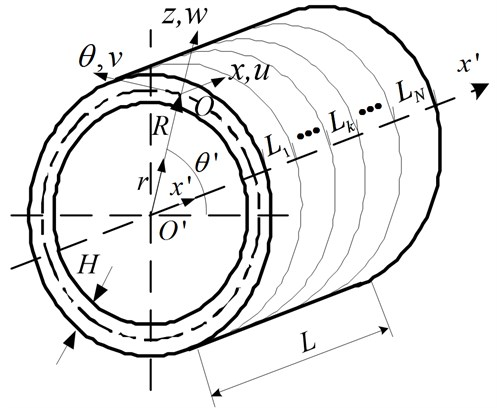The bigger of $N$, the more accurate of the result is, but it spends more time on achieving the result. Thus, the $N$ can’t be too large or too small. As shown in Table 2, the natural frequencies are very close when $N$ is 3, 4 and 5. Considering the time and accuracy, the $N$ is 3 in this paper.

Table 2The natural frequencies with different N (m=1, n=6)

 $N$ 1 2 3 4 5 Natural frequencies 14045.8 1377.44 1369.03 1369.02 1369.02

Based on the Kirchhoff theory, the shear ${V}_{x}$ and transversal shear ${S}_{x}$ are the following :

10
${V}_{x}={Q}_{x}+\frac{1}{R}\frac{\partial {M}_{x\theta }}{\partial \theta },$
11
${S}_{x}={N}_{x\theta }+\frac{1}{R}{M}_{x\theta }.$

Using the Love shell theory, the shell's equilibrium equations are expressed as vibration differential equation :

12
$\begin{array}{l}\frac{\partial {N}_{x}}{\partial s}+\frac{\partial {N}_{x\theta }}{R\partial \theta }-\rho H\frac{{\partial }^{2}u}{\partial {t}^{2}}=0,\\ \frac{\partial {S}_{x}}{\partial x}-\frac{\partial {M}_{x\theta }}{R\partial x}+\frac{\partial {N}_{\theta }}{R\partial \theta }+\frac{{Q}_{\theta }}{R}-\rho H\frac{{\partial }^{2}v}{\partial {t}^{2}}=0,\\ \frac{\partial {V}_{x}}{\partial x}-\frac{\partial {M}_{x\theta }}{R\partial x\partial \theta }+\frac{\partial {Q}_{\theta }}{R\partial \theta }-\frac{{N}_{\theta }}{R}-\rho H\frac{{\partial }^{2}w}{\partial {t}^{2}}=0,\end{array}},$

The relationship of generalized force, corner and middle surface displacement are:

13
${N}_{x}=K\left[\frac{\partial u}{\partial x}+\mu \left(\frac{1}{R}\frac{\partial v}{\partial \theta }+\frac{w}{R}\right)\right],$
14
${M}_{x}=D\left(\frac{\partial {\phi }_{x}}{\partial x}-\frac{\mu }{{R}^{2}}\frac{{\partial }^{2}w}{\partial {\theta }^{2}}\right),$
15
${V}_{x}=\frac{\partial {M}_{x}}{x}-\frac{2}{{R}^{2}}D\left(1-\mu \right)\frac{{\partial }^{3}w}{\partial x\partial {\theta }^{2}},$
16
${S}_{x}=K\frac{1-\mu }{2}\left(\frac{1}{R}\frac{\partial u}{\partial \theta }+\frac{\partial v}{\partial x}\right)-\frac{1}{{R}^{2}}D\left(1-\mu \right)\frac{{\partial }^{2}w}{\partial x\partial \theta },$
17
${\phi }_{x}=-\frac{\partial w}{\partial x},$

where $K$, $D$ are the shell’s membrane stiffness, bending respectively.

The differential equations consist of Eq. (10)-(12), which contain 8 equations and 8 unknown variables. The unknown variables include 3 elastic displacement components, 1 rotation angle and 4 generalized force components. The unknown variables are expressed state vector which are defined as :

18
$\mathbf{Z}\left(x\right)={\left[\begin{array}{cccccccc}u& v& w& {\phi }_{x}& {M}_{x}& {V}_{x}& {S}_{x}& {N}_{x}\end{array}\right]}^{T}.$

Simplified Eq. (12) – Eq. (17) aiming at keeping only the state vector elements yields the following matrix equation:

19
$\frac{\text{d}\mathbf{Z}\left(x\right)}{dx}=\mathbf{U}\mathbf{Z}\left(x\right).$

The coefficient matrices $\mathbf{U}$ of 8×8 order can be expressed in a general form as:

20
$\mathbf{U}=\left[\begin{array}{cccccccc}0& -\frac{\mu }{R}\frac{\partial }{\partial \theta }& -\frac{\mu }{R}& 0& 0& 0& 0& \frac{1-{\mu }^{2}}{Eh}\\ \frac{1}{R}\frac{\partial }{\partial \theta }& 0& 0& -\frac{{h}^{2}}{6{R}^{2}}\frac{\partial }{\partial \theta }& 0& 0& \frac{2\left(1+\mu \right)}{Eh}& 0\\ 0& 0& 0& 1& 0& 0& 0& 0\\ 0& 0& -\frac{\mu }{{R}^{2}}\frac{{\partial }^{2}}{\partial {\theta }^{2}}& 0& \frac{12\left(1-{\mu }^{2}\right)}{E{h}^{3}}& 0& 0& 0\\ 0& 0& 0& -\frac{E{h}^{3}}{6\left(1+\mu \right){R}^{2}}\frac{{\partial }^{2}}{\partial {\theta }^{2}}& 0& -1& 0& 0\\ 0& \frac{Eh}{{R}^{2}}\frac{\partial }{\partial \theta }& \frac{E{h}^{3}}{12{R}^{4}}\frac{{\partial }^{4}}{\partial {\theta }^{4}}+\frac{Eh}{{R}^{2}}+\rho h\frac{{\partial }^{2}}{\partial {t}^{2}}& 0& \frac{\mu }{{R}^{2}}\frac{{\partial }^{2}}{\partial {\theta }^{2}}& 0& 0& \frac{\mu }{R}\\ 0& -\frac{Eh}{{R}^{2}}\frac{{\partial }^{2}}{\partial {\theta }^{2}}+\rho h\frac{{\partial }^{2}}{\partial {t}^{2}}& \frac{E{h}^{3}}{12{R}^{4}}\frac{{\partial }^{3}}{\partial {\theta }^{3}}-\frac{Eh}{{R}^{2}}\frac{\partial }{\partial \theta }& 0& \frac{\mu }{{R}^{2}}\frac{\partial }{\partial \theta }& 0& 0& \frac{\mu }{R}\frac{\partial }{\partial \theta }\\ \rho h\frac{{\partial }^{2}}{\partial {t}^{2}}& 0& 0& -\frac{E{h}^{3}}{12\left(1+\mu \right){R}^{3}}\frac{{\partial }^{2}}{\partial {\theta }^{2}}& 0& 0& -\frac{1}{R}\frac{\partial }{\partial \theta }& 0\end{array}\right].$

According to the geometric characteristics of thin-walled cylindrical shell, the middle surface generalized displacement and generalized force internal force of the short thin cylindrical shell along the $\theta$ direction are written as:

21
$\mathbf{Z}\left(x,\theta ,t\right)=\sum _{m=1}^{\infty }\sum _{n=1}^{\infty }\left[\begin{array}{c}\stackrel{~}{u}\left(x\right)\mathrm{cos}\left(n\theta \right)\\ \stackrel{~}{v}\left(x\right)\mathrm{sin}\left(n\theta \right)\\ \stackrel{~}{w}\left(x\right)\mathrm{cos}\left(n\theta \right)\\ {\stackrel{~}{\phi }}_{x}\left(x\right)\mathrm{cos}\left(n\theta \right)\\ {\stackrel{~}{M}}_{x}\left(x\right)\mathrm{cos}\left(n\theta \right)\\ {\stackrel{~}{V}}_{x}\left(x\right)\mathrm{cos}\left(n\theta \right)\\ {\stackrel{~}{S}}_{x}\left(x\right)\mathrm{sin}\left(n\theta \right)\\ {\stackrel{~}{N}}_{x}\left(x\right)\mathrm{cos}\left(n\theta \right)\end{array}\right]\text{ }{e}^{j\omega t}.$

Substituting Eq. (21) into Eq. (19), the differential equation of modal function can be written as :

22
$\frac{d\stackrel{~}{\mathbf{Z}}\left(x\right)}{dx}=\stackrel{~}{\mathbf{U}}\stackrel{~}{\mathbf{Z}}\left(x\right),$

where $\stackrel{~}{\mathbf{Z}}\left(x\right)={\left[\begin{array}{cccccccc}\stackrel{~}{u}& \stackrel{~}{v}& \stackrel{~}{w}& {\stackrel{~}{\phi }}_{x}& {\stackrel{~}{M}}_{x}& {\stackrel{~}{V}}_{x}& {\stackrel{~}{S}}_{x}& {\stackrel{~}{N}}_{x}\end{array}\right]}^{T}$.

The coefficient matrices $\stackrel{~}{\mathbf{U}}$ of 8×8 order can be expressed in a general form as:

23
$\stackrel{~}{\mathbf{U}}=\left[\begin{array}{cccccccc}0& {U}_{12}& {U}_{13}& 0& 0& 0& 0& {U}_{18}\\ {U}_{21}& 0& 0& {U}_{24}& 0& 0& {U}_{27}& 0\\ 0& 0& 0& {U}_{34}& 0& 0& 0& 0\\ 0& {U}_{42}& {U}_{43}& 0& {U}_{45}& 0& 0& 0\\ {U}_{51}& 0& 0& {U}_{54}& 0& {U}_{56}& {U}_{57}& 0\\ 0& {U}_{62}& {U}_{63}& 0& {U}_{65}& 0& 0& {U}_{68}\\ 0& {U}_{72}& {U}_{73}& 0& {U}_{75}& 0& 0& {U}_{78}\\ {U}_{81}& 0& 0& {U}_{84}& 0& 0& {U}_{87}& 0\end{array}\right].$

Suppose the cylinder is divided into $N$ sections, stress state of any point on the arbitrary cross-section of shell as shown in Figure 3.

Fig. 3Stress state of any point on the arbitrary cross-section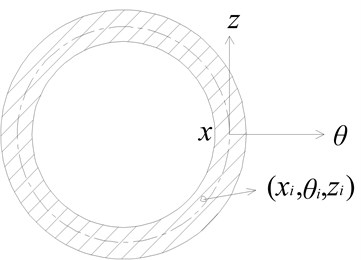According to theory of solving differential equation, the general solution to state equation is the following:

24
$\stackrel{~}{\mathbf{Z}}\left({L}_{k}\right)=\stackrel{~}{\mathbf{G}}\left({L}_{k}\right)\stackrel{~}{\mathbf{Z}}\left({L}_{k-1}\right),$

where ${L}_{k}$ is the length of $k$ th shell segment, $\mathbf{G}$ is transfer matrix and can be written as:

25
$\stackrel{~}{\mathbf{G}}\left({L}_{k}\right)=\mathrm{e}\mathrm{x}\mathrm{p}\left(\stackrel{~}{\mathbf{U}}{L}_{k}\right).$

The modal function can be obtained by reusing transfer matrix and yields the following matrix equation:

26
$\stackrel{~}{\mathbf{Z}}\left(H\right)=\stackrel{~}{\mathbf{T}}\left(\omega \right)\stackrel{~}{\mathbf{Z}}\left(0\right),$

where:

27
$\stackrel{~}{\mathbf{T}}\left(\omega \right)={\stackrel{~}{\mathbf{G}}}_{N}\left({L}_{N}\right){\stackrel{~}{\mathbf{G}}}_{N-1}\left({L}_{N-1}\right)\cdots L{\stackrel{~}{\mathbf{G}}}_{2}\left({L}_{2}\right){\stackrel{~}{\mathbf{G}}}_{1}\left({L}_{1}\right).$

The coefficient matrices $\mathbf{U}$ are obtained by Eq. (22). Using the Eq. (24) and Eq. (26), the transitive relation of the state vectors can be obtained. The transfer matrix $\mathbf{T}$ is 8×8 matrix and can be written by calculating Eq. (27):

28
$\left\{\begin{array}{c}u\\ v\\ w\\ {\phi }_{s}\\ {M}_{s}\\ {V}_{s}\\ {S}_{s}\\ {N}_{s}\end{array}\right\}=⌈\begin{array}{cccccccc}{T}_{11}& {T}_{12}& {T}_{13}& {T}_{14}& {T}_{15}& {T}_{16}& {T}_{17}& {T}_{18}\\ {T}_{21}& {T}_{22}& {T}_{23}& {T}_{24}& {T}_{25}& {T}_{26}& {T}_{27}& {T}_{28}\\ {T}_{31}& {T}_{32}& {T}_{33}& {T}_{34}& {T}_{35}& {T}_{36}& {T}_{37}& {T}_{38}\\ {T}_{41}& {T}_{42}& {T}_{43}& {T}_{44}& {T}_{45}& {T}_{46}& {T}_{47}& {T}_{48}\\ {T}_{51}& {T}_{52}& {T}_{53}& {T}_{54}& {T}_{55}& {T}_{56}& {T}_{57}& {T}_{58}\\ {T}_{61}& {T}_{62}& {T}_{63}& {T}_{64}& {T}_{65}& {T}_{66}& {T}_{67}& {T}_{68}\\ {T}_{71}& {T}_{72}& {T}_{73}& {T}_{74}& {T}_{75}& {T}_{76}& {T}_{77}& {T}_{78}\\ {T}_{81}& {T}_{82}& {T}_{83}& {T}_{84}& {T}_{85}& {T}_{86}& {T}_{87}& {T}_{88}\end{array}⌉\left\{\begin{array}{c}u\\ v\\ w\\ {\phi }_{s}\\ {M}_{s}\\ {V}_{s}\\ {S}_{s}\\ {N}_{s}\end{array}\right\},$

where ${T}_{ij}\left(i=1,2,\cdots ,8;j=1,2,\cdots ,8\right)$ are the transfer matrix coefficients.

### 3.2. Analytical method

The transfer matrixes are different on the different boundary conditions. The transfer matrixes are given as follows for the following boundary condition, and then the natural frequencies can be obtained.

a. Clamped-clamped

Set $s=0$, $u=v=w={\phi }_{x}=0$;

Set $s=L$, $u=v=w={\phi }_{x}=0$.

Substitute Eq. (29) into Eq. (28), Eq. (29) are expressed as following:

29
${\mathbf{T}}^{\text{'}}=\left[\begin{array}{cccc}{T}_{15}& {T}_{16}& {T}_{17}& {T}_{18}\\ {T}_{25}& {T}_{26}& {T}_{27}& {T}_{28}\\ {T}_{35}& {T}_{36}& {T}_{37}& {T}_{38}\\ {T}_{45}& {T}_{46}& {T}_{47}& {T}_{48}\end{array}\right].$

The determinant of the coefficients of the matrix ${\mathbf{Τ}}^{\mathbf{\text{'}}}$ is zero:

30
$\mathrm{D}\mathrm{e}\mathrm{t}\left({\mathbf{Τ}}^{\mathbf{\text{'}}}\right)=0.$

The matrix ${\mathbf{Τ}}^{\mathbf{\text{'}}}$ is the function of natural frequencies. So the natural frequencies corresponding to modal are obtained.

b. Clamped-free

Set $s=0$, $u=v=w={\phi }_{x}=0$;

Set $s=L$, ${M}_{x}={N}_{x}={V}_{x}={S}_{x}=0$;

31
${\mathbf{T}}^{\text{'}}=\left[\begin{array}{cccc}{T}_{11}& {T}_{12}& {T}_{13}& {T}_{14}\\ {T}_{21}& {T}_{22}& {T}_{23}& {T}_{24}\\ {T}_{31}& {T}_{32}& {T}_{33}& {T}_{34}\\ {T}_{41}& {T}_{42}& {T}_{43}& {T}_{44}\end{array}\right].$

The determinant of the coefficients of the matrix ${\mathbf{Τ}}^{\mathbf{\text{'}}}$ is zero. So the natural frequencies corresponding to modal are obtained.

## 4. Numerical computation based on finite element method

In the classical vibration theory, the basic equation of dynamic problems based on finite element methods is presented as follows :

32
$\mathbf{M}\stackrel{¨}{\mathbf{\delta }}\left(t\right)+\mathbf{C}\stackrel{˙}{\mathbf{\delta }}\left(t\right)+\mathbf{K}\mathbf{\delta }\left(t\right)=\mathbf{F},$

where $\mathbf{M}$ is the total mass matrix, $\mathbf{C}$ is the total damping matrix, $\mathbf{K}$ is the total stiffness matrix, $\mathbf{F}$ is the total additional exciting force matrix, $\mathbf{\delta }$, $\stackrel{˙}{\mathbf{\delta }}$ and $\stackrel{¨}{\mathbf{\delta }}$ are the joint displacement, velocity and acceleration column matrixes respectively.

As the natural vibration frequency of structural system is calculated with the consideration that the free vibration is conducted under undamped conditions. The equation of the natural vibration frequency is expressed as:

33
$\mathbf{M}\stackrel{¨}{\mathbf{\delta }}\left(t\right)+\mathbf{K}\mathbf{\delta }\left(t\right)=0.$

The solutions can be presumed as the following types:

34
$\mathbf{\delta }=\mathbf{\phi }\mathrm{s}\mathrm{i}\mathrm{n}\omega \left(t-{t}_{0}\right),$

where $\phi$ is the $n$ order vector quantity, $\omega$ is the natural frequency.

The Eq. (33) is substituted into Eq. (34), and then generalized eigenvalue problems are obtained as follows:

35
$\mathbf{K}\mathbf{\phi }-{\omega }^{2}\mathbf{M}\mathbf{\phi }=0,\text{or}\left[\mathbf{K}-{\omega }^{2}\mathbf{M}\right]\mathbf{\phi }=0.$

According to the linear algebra theory, the necessary and sufficient conditions which allow Eq. (35) with untrivial solution are as follows:

36
$\mathrm{d}\mathrm{e}\mathrm{t}\left(\mathbf{K}-{\omega }^{2}\mathbf{M}\right)\text{=}0,$

where $n$ characteristic solutions can be obtained by solving these equations, such as$\left({\omega }_{1}^{2},{\phi }_{1}\right),\left({\omega }_{2}^{2},{\phi }_{2}\right),\dots ,\left({\omega }_{n}^{2},{\phi }_{n}\right),$ among which ${\omega }_{1},{\omega }_{2},\dots ,\mathrm{}{\omega }_{n}$ stand for $n$ natural frequencies of system, and correspond to $0\le {\omega }_{1}<{\omega }_{2}<\cdot \cdot \cdot <{\omega }_{n}$.

For every natural frequencies of structure, relative amplitudes of each node from one group can be concluded based on Eq. (35). Eigenvectors such as ${\mathbf{\phi }}_{1},{\mathbf{\phi }}_{2},\dots ,{\mathbf{\phi }}_{n}$ represent $n$ natural modes of vibration of structure. Their amplitudes can be set as follows:

37
${\mathbf{\phi }}_{i}^{T}\mathbf{M}{\mathbf{\phi }}_{i}=1\text{ }\left(i=\text{1},\text{2},\text{3},\dots ,n\right).$

Natural mode of vibration is orthogonal compared to matrix $\mathbf{M}$. Natural mode of vibration is defined as:

38
$\mathbf{\Phi }=\left[\begin{array}{cccc}{\mathbf{\phi }}_{1}& {\mathbf{\phi }}_{2}& \dots & {\mathbf{\phi }}_{n}\end{array}\right].$

Then it can be obtained as follows:

39
${\mathbf{\Omega }}^{2}=\text{diag}\left(\begin{array}{cccc}{\omega }_{1}^{2}& {\omega }_{2}^{2}& \dots & {\omega }_{n}^{2}\end{array}\right).$

The characters of characteristic solution can be also expressed as:

40
${\mathbf{\Phi }}^{T}\mathbf{\Omega }\mathbf{\Phi }=\mathbf{I},\text{\hspace{0.17em}\hspace{0.17em}\hspace{0.17em}\hspace{0.17em}\hspace{0.17em}}{\mathbf{\Phi }}^{T}\mathbf{K}\mathbf{\Phi }={\mathbf{\Omega }}^{2},$

where $\mathbf{\Phi }$ and ${\mathbf{\Omega }}^{2}$ are the natural mode of vibration matrix and natural frequency matrix respectively.

## 5. The results of the three analytical methods

The natural frequencies of the static state of the cylindrical shell under clamped – free and clamped boundary conditions are calculated by the transfer matrix method, the beam function method and the finite element method, respectively, and make further verification of the natural frequencies. Table 3 shows the basic parameters of the thin-walled cylindrical shell.

Table 3The basic parameters of the thin-walled cylindrical shell

 Youngmodulus$E$ (Pa) Poisson'sratio$\mu$ Density$\rho$(kg/m3) Length$L$(mm) Thickness$H$(mm) Insideradius$R$ (mm) Externalradius$R$ (mm) Material 2.12×1011 0.3 7850 95 2 142 144 Structural steel

Table 4The first 20 order natural frequencies in the clamped – free boundary conditions (Unit: Hz)

 Order 1 order 2 order 3 order 4 order 5 order Modal shape description $m=$ 1, $n=$ 6 $m=$ 1, $n=$ 5 $m=$ 1, $n=$ 7 $m=$ 1, $n=$ 4 $m=$ 1, $n=$ 8 Transfer matrix 1369 1424 1500 1701 1768 Beam function 1717 1858 1734 2168 1893 Finite element 1380 1450 1497 1739 1756 Order 6 order 7 order 8 order 9 order 10 order Modal shape description $m=$ 1, $n=$ 9 $m=$ 1, $n=$ 3 $m=$ 1, $n=$ 10 $m=$ 1, $n=$ 11 $m=$ 1, $n=$ 2 Transfer matrix 2131 2230 2567 3062 3091 Beam function 2174 2701 2552 3010 3636 Finite element 2115 2274 2550 3048 3137 Order 11 order 12 order 13 order 14 order 15 order Modal shape description $m=$ 1, $n=$ 12 $m=$ 2, $n=$ 7 $m=$ 2, $n=$ 8 $m=$ 2, $n=$ 6 $m=$ 2, $n=$ 9 Transfer matrix 3612 3611 3633 3752 3801 Beam function 3536 3369 3411 3457 3572 Finite element 3607 3686 3721 3818 3909 Order 16 order 17 order 18 order 19 order 20 order Modal shape description $m=$ 2, $n=$ 5 $m=$ 1, $n=$ 13 $m=$ 2, $n=$ 10 $m=$ 1, $n=$1 $m=$ 2, $n=$ 4 Transfer matrix 4062 4215 4092 4437 4519 Beam function 3683 4124 3841 4935 4062 Finite element 4118 4223 4225 4476 4564

The natural frequencies of the cylindrical shell under clamped – free are calculated by the transfer matrix method, the beam function method and the finite element method respectively. Table 4 and Fig. 4 shows that the results of the transfer matrix method and the finite element method calculation are very close, the result of beam function method calculation is close to those of transfer matrix method and the finite element method calculation only at the place of 6th order, the 8th order, the 9th order and the 11th order, the frequencies of other orders are quite different.

The natural frequencies of the cylindrical shell under both ends clamped boundary conditions are calculated by the transfer matrix method, the beam function method and the finite element method, respectively. Table 5 and Fig. 5 shows that the results of transfer matrix method and the finite element method calculation are very close, the result of beam function method calculation is close to those of transfer matrix method and the finite element method calculation only at the place of 8th order, the 10th order and the 12th order, the frequencies of other orders are quite different.

Fig. 4The frequencies comparison of the three methods in the clamped – free boundary condition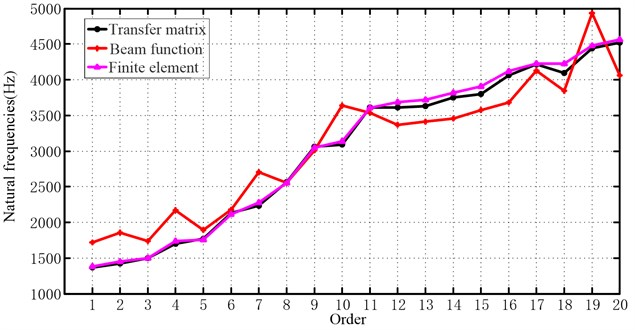Table 5The first 20 order natural frequencies in the both ends clamped boundary condition (Unit: Hz)

 Order 1 order 2 order 3 order 4 order 5 order Modal shape description $m=$ 1, $n=$ 7 $m=$ 1, $n=$ 8 $m=$ 1, $n=$ 6 $m=$ 1, $n=$ 9 $m=$ 1, $n=$ 5 Transfer matrix 3051 3105 3136 3285 3369 Beam function 3239 3282 3332 3449 3572 Finite element 3115 3172 3198 3358 3428 Order 6 order 7 order 8 order 9 order 10 order Modal shape description $m=$ 1, $n=$ 10 $m=$ 1, $n=$ 4 $m=$ 1, $n=$ 11 $m=$ 1, $n=$ 3 $m=$ 1, $n=$ 12 Transfer matrix 3576 3761 3964 4322 4435 Beam function 3726 3967 4100 4522 4559 Finite element 3657 3816 4059 4368 4547 Order 11 order 12 order 13 order 14 order 15 order Modal shape description $m=$ 1, $n=$ 2 $m=$ 1, $n=$ 13 $m=$ 1, $n=$ 1 $m=$ 1, $n=$ 14 $m=$ 2, $n=$ 7 Transfer matrix 5034 4981 5759 5592 5804 Beam function 5209 5094 5881 5696 5696 Finite element 5066 5126 5770 5774 5943 Order 16 order 17 order 18 order 19 order 20 order Modal shape description $m=$ 2, $n=$ 6 $m=$ 2, $n=$ 8 $m=$ 2, $n=$ 5 $m=$ 1, $n=$0 $m=$ 2, $n=$ 9 Transfer matrix 5862 5836 5996 6103 5963 Beam function 5930 5929 6059 6189 6067 Finite element 5974 6012 6086 6101 6179

Fig. 5The frequencies comparison of the three methods in the both ends clamped boundary condition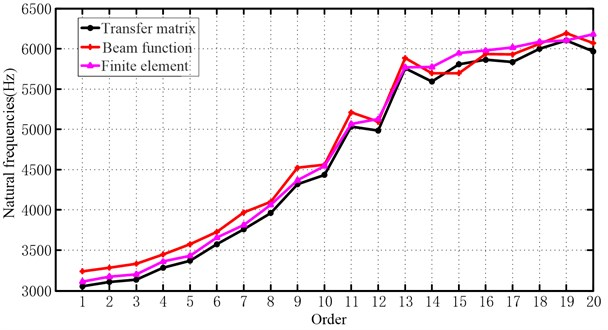## 6. Experimental test and comparison

A method for identification the natural frequencies and the mode shape of cantilever circular cylindrical shells is presented, when striking it with a hammer. The hammering test system is shown in Fig. 6, they are impact measurement system consisting of modal hammer, polytec, LMS measurement system and a high-performance computer and so on.

Fig. 6Impact measurement system for modal of cantilever circular cylindrical shells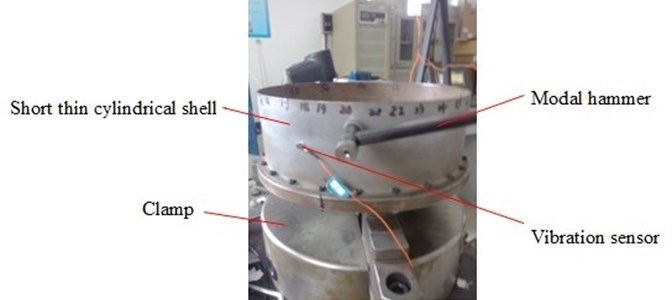Table 6The results of experiments and finite element method

 Order Modal shape description Experiment Finite element Difference (%) 1 1,6 1065.3 1380 22.80 2 1,7 1310.6 1497 12.45 3 1,8 1627.9 1756 7.29 4 1,9 2005.6 2115 5.17 5 1,10 2436.1 2550 4.47 6 1,11 2916.0 3048 4.33

The natural characteristics are obtained by the way of multi-point excitation and a single point response, the drum is divided into five laps, and 36 points per circle, the sensors are fixed in the point (lap 10th point), an incentive hammer hammers sequentially 5×36 points.

The results are shown in Table 6 and Fig. 7. The results of the transfer matrix method and the finite element method are close to that of experiments, while the results vary wildly between the transfer matrix method and the beam functions method, however the operation time of the beam functions method is short, and its calculation process is simple.

Fig. 7Three algorithms compared to experimental results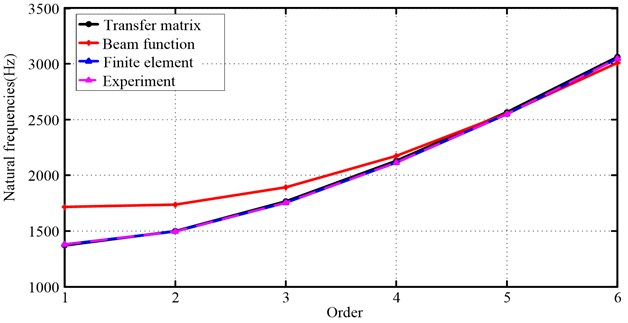## 7. Conclusions

In this paper, analytical method (beam functions method), semi-analytical method (transfer matrix method) and numerical methods (finite element method) are presented to solve the natural characteristics of thin-walled cylindrical shell. The results in the clamped-free and clamped-clamped boundary conditions are focused on comparison. Furthermore, the experiments of the short thin cylindrical shell in the clamped-free boundary conditions are studied, which demonstrated that:

(1) Results of transfer matrix method and finite element method are basically similar, while great difference is obtained by beam functions method.

(2) Further studies by experiment have confirmed that the results obtained by transfer matrix method and finite element method are more accurate and efficient.

(3) Due to the influence of factors such as geometrical dimension, material parameters, machining accuracy and chucking ways, the biases between experimental results and calculation results is inevitable, but identical trends are observed during the study.

10 May 2013
Accepted
04 September 2013
Published
30 September 2013
Keywords
short thin cylindrical shell
vibration characteristics
natural frequencies
beam functions method
transfer matrix method
finite element method
Acknowledgements

This work is supported by National Science Foundation of China (51105064), National Program on Key Basic Research Project (2012CB026000), and Natural Science Foundation of Liaoning Province (201202056).